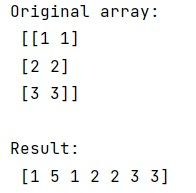# Prepend element to numpy array

Learn, how to prepend element to numpy array in Python?
Submitted by Pranit Sharma, on January 14, 2023

NumPy is an abbreviated form of Numerical Python. It is used for different types of scientific operations in python. Numpy is a vast library in python which is used for almost every kind of scientific or mathematical operation. It is itself an array which is a collection of various methods and functions for processing the arrays.

## Prepending element to numpy array

Suppose we are given a NumPy array and we need to insert some element at the beginning of this array.

For this purpose, we will use numpy.insert() method. This method inserts values along the given axis before the given indices.

If the type of values is different from that of arr, values are converted to the type of arr.Values.

It takes an array as an argument in which the element has to be inserted and it takes an argument obj which is the object that defines the index or indices before which values are inserted.
It returns a copy of arr with values inserted. Note that insert does not occur in-place: a new array is returned. If the axis is None, the output is a flattened array.

Let us understand with the help of an example,

## Python code to prepend element to numpy array

```# Import numpy
import numpy as np

# Creating a numpy array
arr = np.array([[1, 1], [2, 2], [3, 3]])

# Display original array
print("Original array:\n",arr,"\n")

# Inserting 5 at 1st position
arr = np.insert(arr, 1, 5)

# Display result
print("Result:\n",arr)
```

Output:What's New (MCQs)

Top Interview Coding Problems/Challenges!

IncludeHelp's Blogs

Languages: » C » C++ » C++ STL » Java » Data Structure » C#.Net » Android » Kotlin » SQL
Web Technologies: » PHP » Python » JavaScript » CSS » Ajax » Node.js » Web programming/HTML
Solved programs: » C » C++ » DS » Java » C#
Aptitude que. & ans.: » C » C++ » Java » DBMS
Interview que. & ans.: » C » Embedded C » Java » SEO » HR
CS Subjects: » CS Basics » O.S. » Networks » DBMS » Embedded Systems » Cloud Computing
» Machine learning » CS Organizations » Linux » DOS
More: » Articles » Puzzles » News/Updates